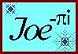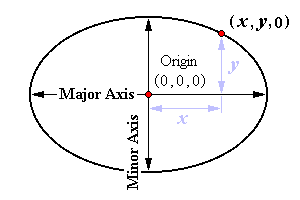Elliptic Roof Calculator Scope: 1st and 2nd Quadrants
 Enter Ellipse Dimensions
 Major Axis: x-axis Minor Axis: y-axis
 Define height ( 0, 0, z ) at Center
 Rise, z =
 Define first endpoint of Chord
 ( x1 > x2 )  x1 =
 Define second endpoint of Chord
 ( x2 < x1 )  x2 =
 HIP #1 Data ( 0, 0, z ) - ( x1, y1, 0 )
x1 =
y1 =
Hip 1 Run =
Hip 1 Length =
wrt to x-axis Deck θ =
Hip 1 Pitch θ =
Hip 1 Deck θ =
Hip 1 Backing θ =
Hip 1 Roof Plane θ =
 HIP #2 Data ( 0, 0, z ) - ( x2, y2, 0 )
x2 =
y2 =
Hip 2 Run =
Hip 2 Length =
wrt to x-axis Deck θ =
Hip 2 Pitch θ =
Hip 2 Deck θ =
Hip 2 Backing θ =
Hip 2 Roof Plane θ =
 Chord-Common Rafter Data ( x1, y1, 0 ) - ( x2, y2, 0 )
x1 =
y1 =
x2 =
y2 =
Δx: x1 - x2 =
Δy: y1 - y2 =
Chord Length =
Common Pitch θ =
at (0, 0, 0) Deck θ =
at peak Roof Plane θ =Elliptic Roof Calculator Entries Elliptic Roof Calculator Images

 Cartesian Equation Data
x ² coefficient =
y ² coefficient =
Constant =
 Feet to Inch - Frac. Inch Select Denominator Value Click on Dimension Field at left
Fraction :
Length :

 Inches :
 Angle to Rise / Run Select Denominator Value Click on Angle Field at left Scope: 0° < θ < 90°
Fraction :
Degrees :
Over ? :

 Inches :